Home
Hostname: page-component-cf9d5c678-dkwk2 Total loading time: 0.188 Render date: 2021-07-29T18:12:49.302Z Has data issue: true Feature Flags: { "shouldUseShareProductTool": true, "shouldUseHypothesis": true, "isUnsiloEnabled": true, "metricsAbstractViews": false, "figures": true, "newCiteModal": false, "newCitedByModal": true, "newEcommerce": true, "newUsageEvents": true }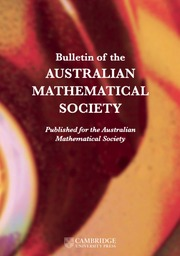Bulletin of the Australian Mathematical Society

## Abstract

A subset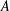$A$ of a finite abelian group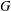$G$ is called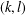$(k,l)$-sum-free if the sum of$k$ (not necessarily distinct) elements of$A$ never equals the sum of$l$ (not necessarily distinct) elements of$A$. We find an explicit formula for the maximum size of a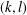$(k,l)$-sum-free subset in$G$ for all$k$ and$l$ in the case when$G$ is cyclic by proving that it suffices to consider$(k,l)$-sum-free intervals in subgroups of$G$. This simplifies and extends earlier results by Hamidoune and Plagne [‘A new critical pair theorem applied to sum-free sets in abelian groups’, Comment. Math. Helv. 79(1) (2004), 183–207] and Bajnok [‘On the maximum size of a$(k,l)$-sum-free subset of an abelian group’, Int. J. Number Theory 5(6) (2009), 953–971].

## MSC classification

Type
Research Article
Information
© 2018 Australian Mathematical Publishing Association Inc.

## Access options

Get access to the full version of this content by using one of the access options below. (Log in options will check for institutional or personal access. Content may require purchase if you do not have access.)

## References

Bajnok, B., ‘On the maximum size of a (k, l)-sum-free subset of an abelian group’, Int. J. Number Theory 5(6) (2009), 953971.CrossRefGoogle Scholar
Balasubramanian, R., Prakash, G. and Ramana, D. S., ‘Sum-free subsets of finite abelian groups of type III’, European J. Combin. 58 (2016), 181202.CrossRefGoogle Scholar
Bier, T. and Chin, A. Y. M., ‘On (k, l)-sets in cyclic groups of odd prime order’, Bull. Aust. Math. Soc. 63(1) (2001), 115121.CrossRefGoogle Scholar
Chervak, O., Pikhurko, O. and Staden, K., ‘Minimum number of additive tuples in groups of prime order’, Preprint, 2017, arXiv:1710.01936 [math.CO].Google Scholar
Diananda, P. H. and Yap, H. P., ‘Maximal sum-free sets of elements of finite groups’, Proc. Japan Acad. 45 (1969), 15.CrossRefGoogle Scholar
Erdős, P., ‘Extremal problems in number theory’, in: Proc. Sympos. Pure Mathematics, Vol. VIII (American Mathematical Society, Providence, RI, 1965), 181189.Google Scholar
Green, B. and Ruzsa, I., ‘Sum-free sets in abelian groups’, Israel J. Math. 147 (2005), 157188.CrossRefGoogle Scholar
Hamidoune, Y. O. and Plagne, A., ‘A new critical pair theorem applied to sum-free sets in abelian groups’, Comment. Math. Helv. 79(1) (2004), 183207.CrossRefGoogle Scholar
Plagne, A., ‘Maximal (k, l)-free sets in ℤ/pℤ are arithmetic progressions’, Bull. Aust. Math. Soc. 65(3) (2002), 137144.CrossRefGoogle Scholar
Samotij, W. and Sudakov, B., ‘The number of additive triples in subsets of abelian groups’, Math. Proc. Cambridge Philos. Soc. 160(3) (2016), 495512.CrossRefGoogle Scholar
Samotij, W. and Sudakov, B., ‘The number of additive triples in subsets of abelian groups’, Preprint, 2015, arXiv:1507.03764 [math.NT].Google Scholar
Street, A. P., ‘Maximal sum-free sets in abelian groups of order divisible by three’, Bull. Aust. Math. Soc. 6 (1972), 439441.CrossRefGoogle Scholar
Street, A. P., ‘Corrigendum: “Maximal sum-free sets in abelian groups of order divisible by three”’, Bull. Aust. Math. Soc. 7 (1972), 317318.CrossRefGoogle Scholar
Wallis, W. D., Street, A. P. and Wallis, J. S., Combinatorics: Room Squares, Sum-free Sets, Hadamard Matrices, Lecture Notes in Mathematics, 292 (Springer, Berlin–New York, 1972).CrossRefGoogle Scholar
Yap, H. P., ‘Maximal sum-free sets in finite abelian groups. II’, Bull. Aust. Math. Soc. 5 (1971), 4354.CrossRefGoogle Scholar

# Send article to Kindle

Note you can select to send to either the @free.kindle.com or @kindle.com variations. ‘@free.kindle.com’ emails are free but can only be sent to your device when it is connected to wi-fi. ‘@kindle.com’ emails can be delivered even when you are not connected to wi-fi, but note that service fees apply.

Find out more about the Kindle Personal Document Service.

THE MAXIMUM SIZE OF$(k,l)$-SUM-FREE SETS IN CYCLIC GROUPS Available formats × # Send article to Dropbox To send this article to your Dropbox account, please select one or more formats and confirm that you agree to abide by our usage policies. If this is the first time you use this feature, you will be asked to authorise Cambridge Core to connect with your <service> account. Find out more about sending content to Dropbox. THE MAXIMUM SIZE OF$(k,l)$-SUM-FREE SETS IN CYCLIC GROUPS
Available formats
×

# Send article to Google Drive

To send this article to your Google Drive account, please select one or more formats and confirm that you agree to abide by our usage policies. If this is the first time you use this feature, you will be asked to authorise Cambridge Core to connect with your <service> account. Find out more about sending content to Google Drive.

THE MAXIMUM SIZE OF$(k,l)\$-SUM-FREE SETS IN CYCLIC GROUPS
Available formats
×
×